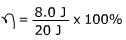# Efficiency Formula

Efficiency Formula

Efficiency is a measure of how much work or energy is conserved in a process. In many processes, work or energy is lost, for example as waste heat or vibration. The efficiency is the energy output, divided by the energy input, and expressed as a percentage. A perfect process would have an efficiency of 100%.η = efficiency (Greek letter "eta")

Wout = the work or energy produced by a process. Units are Joules (J).

Win = the work or energy put in to a process. Units are Joules (J).

Efficiency Formula Questions:

1) A construction worker puts 20 J of energy in to one strike of his hammer on the head of a nail. The energy transferred to driving the nail in to the wood is 8.0 J. What is the efficiency of the construction worker's hammering?

Answer: The efficiency can be found using the formula:η = 0.40 x 100%

η = 40%

The efficiency of the hammer strike was 40%. Vibrations and heating of the nail are two possible reasons for the energy loss.

2) A particular chemical process has an energy efficiency of only 3.00%. To complete this large-scale chemical process, 140,000 J of energy is input. What is the energy output of this process?

Answer: The energy output can be found by rearranging the formula for efficiency:Wout = (140,000 J)(0.03)

Wout = 4,200 J

The energy output of this chemical process is 4,200 J.

 Related Links: Efficiency Quiz